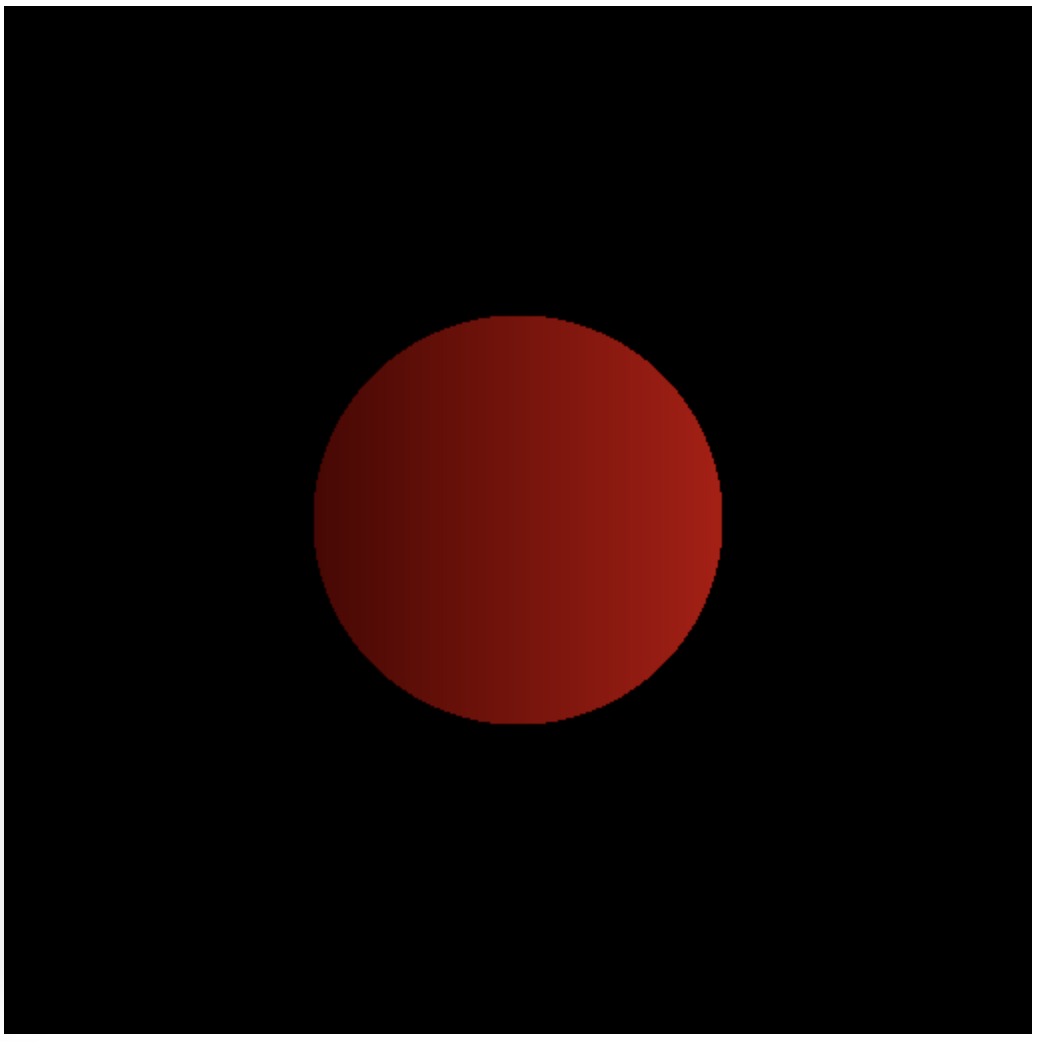Abubu.js
The WebGL Computational Library## Draw a circle using the fragment shader

We can modify the fragment shader that we designed in the previous example to do more complex things than just coloring a gradient.

In this example, we modify the shader to draw only a circle at the center of the canvas using the default geometry and vertex shader.In order to do this, all we have to do is to add a conditional to our fragment shader. So, the new shader can be implemented as

#version 300 es
precision highp float ;
precision highp int ;

out vec4 outcolor ; /*  output of the shader
pixel color         */
in vec2 cc ;        /* input from vertex shader */

// Main body of the shader
void main() {
/* Check if the pixel is inside the circle
and color it with a gradient. Otherwise, color it
black   */
if ( length(cc-vec2(0.5,0.5)) < 0.2){
outcolor = vec4(cc.x,0.,0.,1.) ;
}else{
outcolor = vec4(0.,0.,0.,1.) ;
}
return ;
}


The result will be a page that produces the shape below.The circle drawing program

### What is the geometry that is being drawn?

One would think that we are drawing a circle. However, what the WebGL 2.0 is actually drawing is just a geometry made of two triangles! The triangular surfaces are colored in the fragment shader such that you see a circle on top of them!

Download the source code for all tutorials